• 本文想说的是折线点的地方显示数据纵坐标数据 利用：plt.text() 方法 废话不多说，直接上代码： # encoding=utf-8 import matplotlib.pyplot as plt # 月份 x1 = ['2017-01', '2017-02', '2017-03', '2017-04...
本文想说的是折线点的地方显示数据纵坐标数据
利用：plt.text() 方法
废话不多说，直接上代码：

# encoding=utf-8
import matplotlib.pyplot as plt

# 月份
x1 = ['2017-01', '2017-02', '2017-03', '2017-04', '2017-05', '2017-06', '2017-07', '2017-08',
'2017-09', '2017-10', '2017-11', '2017-12']

# 体重
y1 = [86, 85, 84, 80, 75, 70, 70, 74, 78, 70, 74, 80]

# 设置画布大小
plt.figure(figsize=(16, 4))

# 标题
plt.title("my weight")

# 数据
plt.plot(x1, y1, label='weight changes', linewidth=3, color='r', marker='o',
markerfacecolor='blue', markersize=20)

# 横坐标描述
plt.xlabel('month')

# 纵坐标描述
plt.ylabel('weight')

# 设置数字标签
for a, b in zip(x1, y1):
plt.text(a, b, b, ha='center', va='bottom', fontsize=20)

plt.legend()
plt.show()

效果：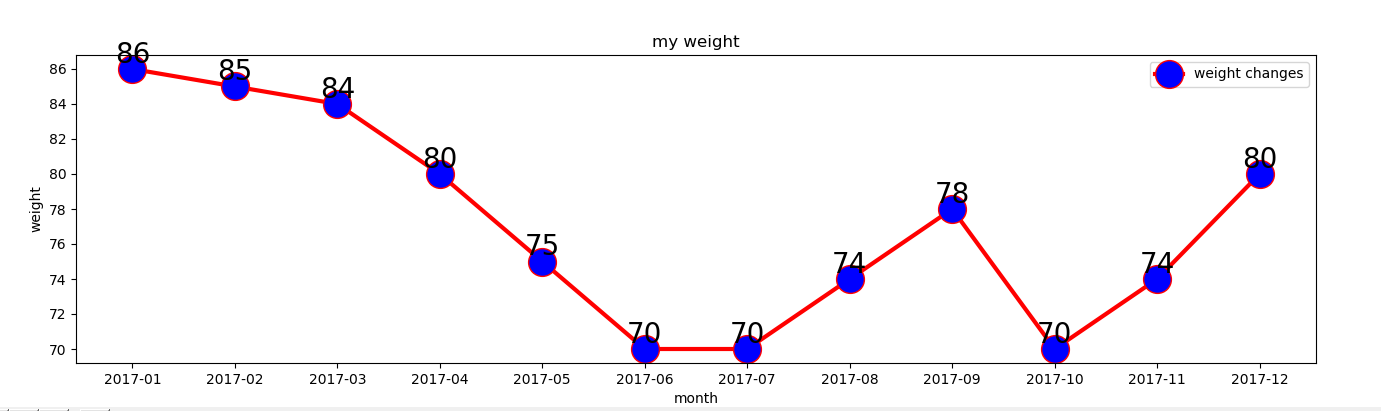展开全文matplotlib
• 主要一个股票，使用时间序列模型研究按照下面的流程来研究一下其变化趋势，看看准不准，Python代码写的比较优美，学习者可以研读一下整个程序流程。 步骤： 准备数据 可视化数据、审查数据 处理数据 根据...
前言
本文的文字及图片来源于网络,仅供学习、交流使用,不具有任何商业用途,如有问题请及时联系我们以作处理。
以下文章来源于数据皮皮侠，作者：皮皮侠
主要一个股票，使用时间序列模型研究按照下面的流程来研究一下其变化趋势，看看准不准，Python代码写的比较优美，学习者可以研读一下整个程序流程。步骤：
准备数据可视化数据、审查数据处理数据根据ACF、PACF定阶拟合ARIMA模型预测
# -*- coding: utf-8 -*-
"""
Spyder Editor

This is a temporary script file.
"""

import pandas as pd
import datetime
import matplotlib.pylab as plt
from matplotlib.pylab import style
from statsmodels.tsa.arima_model import ARIMA
from statsmodels.graphics.tsaplots import plot_acf, plot_pacf

style.use('ggplot')     # 设置图片显示的主题样式

# 解决matplotlib显示中文问题
plt.rcParams['font.sans-serif'] = ['SimHei']  # 指定默认字体
plt.rcParams['axes.unicode_minus'] = False  # 解决保存图像是负号'-'显示为方块的问题

def run_main():
"""
主函数
"""
# 1. 准备数据
# 指定股票分析开始日期
start_date = datetime.datetime(2009, 1, 1)
# 指定股票分析截止日期
end_date = datetime.datetime(2019, 4, 1)
# 股票代码
stock_code = '600519.SS'    # 沪市贵州茅台

stock_code, 'yahoo', start_date, end_date
)
# 预览数据

# 2. 可视化数据
plt.plot(stock_df['Close'])
plt.title('股票每日收盘价')
plt.show()

# 按周重采样
stock_s = stock_df['Close'].resample('W-MON').mean()
stock_train = stock_s['2014':'2018']
plt.plot(stock_train)
plt.title('股票周收盘价均值')
plt.show()

# 分析 ACF
acf = plot_acf(stock_train, lags=20)
plt.title("股票指数的 ACF")
acf.show()

# 分析 PACF
pacf = plot_pacf(stock_train, lags=20)
plt.title("股票指数的 PACF")
pacf.show()

# 3. 处理数据，平稳化数据
# 这里只是简单第做了一节差分，还有其他平稳化时间序列的方法
stock_diff = stock_train.diff()
diff = stock_diff.dropna()
print(diff.dtypes)

plt.figure()
plt.plot(diff)
plt.title('一阶差分')
plt.show()

acf_diff = plot_acf(diff, lags=20)
plt.title("一阶差分的 ACF")
acf_diff.show()

pacf_diff = plot_pacf(diff, lags=20)
plt.title("一阶差分的 PACF")
pacf_diff.show()

# 4. 根据ACF和PACF定阶并建立模型
model = ARIMA(stock_train, order=(1, 1, 1), freq='W-MON')
# 拟合模型
arima_result = model.fit()
print(arima_result.summary())

# 5. 预测

pred_vals = arima_result.predict(start=str('2019-01'),end=str('2019-03'),
dynamic=False, typ='levels')
print(pred_vals)

# 6. 可视化预测结果
stock_forcast = pd.concat([stock_s, pred_vals], axis=1, keys=['original', 'predicted'])

plt.figure()
plt.plot(stock_forcast)
plt.title('真实值vs预测值')
plt.savefig('./stock_pred.png', format='png')
plt.show()

if __name__ == '__main__':
run_main()

结果显示：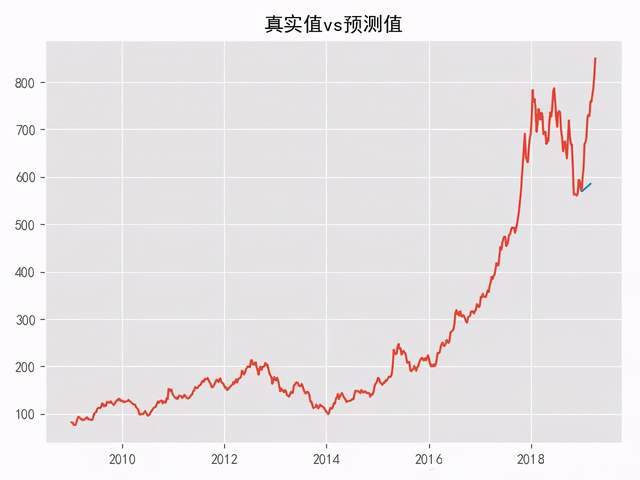展开全文可视化
• 1. 用 Numpy ndarray 作为数据传入 plyimport numpy as npimport matplotlib as mplimport matplotlib.pyplot as pltnp.random.seed(1000)y = np.random.standard_normal(10)print "y = %s"% yx = range...
1. 用 Numpy ndarray 作为数据传入 ply
import numpy as np
import matplotlib as mpl
import matplotlib.pyplot as plt
np.random.seed(1000)
y = np.random.standard_normal(10)
print "y = %s"% y
x = range(len(y))
print "x=%s"% x
plt.plot(y)
plt.show()2. 操纵坐标轴和增加网格及标签的函数
import numpy as np
import matplotlib as mpl
import matplotlib.pyplot as plt
np.random.seed(1000)
y = np.random.standard_normal(10)
plt.plot(y.cumsum())
plt.grid(True) ##增加格点
plt.axis('tight') # 坐标轴适应数据量 axis 设置坐标轴
plt.show()3. plt.xlim 和 plt.ylim 设置每个坐标轴的最小值和最大值
#!/etc/bin/python
#coding=utf-8
import numpy as np
import matplotlib as mpl
import matplotlib.pyplot as plt
np.random.seed(1000)
y = np.random.standard_normal(20)
plt.plot(y.cumsum())
plt.grid(True) ##增加格点
plt.xlim(-1,20)
plt.ylim(np.min(y.cumsum())- 1, np.max(y.cumsum()) + 1)
plt.show()4. 添加标题和标签 plt.title, plt.xlabe, plt.ylabel 离散点, 线
#!/etc/bin/python
#coding=utf-8
import numpy as np
import matplotlib as mpl
import matplotlib.pyplot as plt
np.random.seed(1000)
y = np.random.standard_normal(20)
plt.figure(figsize=(7,4)) #画布大小
plt.plot(y.cumsum(),'b',lw = 1.5) # 蓝色的线
plt.plot(y.cumsum(),'ro') #离散的点
plt.grid(True)
plt.axis('tight')
plt.xlabel('index')
plt.ylabel('value')
plt.title('A simple Plot')
plt.show()以上就是Python 绘制可视化折线图的详细内容，更多关于Python 绘制折线图的资料请关注python博客其它相关文章！
展开全文• matplotlib是Python的一个强大的2D图形绘制库，使用需要安装奥，通过调用它内部的函数接口，可以清爽的生成直方、饼状、功率谱、条形、错误、散点等等 matplotlib安装。 pipinstallmatplotlib 点击...
前言
本文的文字及图片来源于网络,仅供学习、交流使用,不具有任何商业用途,版权归原作者所有,如有问题请及时联系我们以作处理。
matplotlib是Python的一个强大的2D图形绘制库，使用需要安装奥，通过调用它内部的函数接口，可以清爽的生成直方图、饼状图、功率谱、条形图、错误图、散点图等等
matplotlib安装。
pip install matplotlib

点击加群即可免费获取Python资料
资料就放在群文件里等你来拿哟

实例代码
不废话了，说明都在注释里了！！！
import pandas as pd
import matplotlib.pyplot as mpl               # 引入matplotlib库

# 用来正常显示中文标签
mpl.rcParams['font.sans-serif'] = ['SimHei']

# 定义x、y轴列表数据缓冲
x_axes=[]
y_axes=[]

# 读取表格信息

# x和y轴数据，取excel单号列和数量列
x_axes = sheet['单号']
y_axes = sheet['数量']

""" 1折线图 """

mpl.plot(x_axes,y_axes,label='单号数量折线图')

# 设置标题及横纵坐标名称
mpl.title('单号数量关系')
mpl.xlabel(u'单号')
mpl.ylabel(u'数量')

# 显示图表
mpl.show()

""" 2条形图 """

mpl.title('单号数量关系')
mpl.xlabel(u'单号')
mpl.ylabel(u'数量')

mpl.bar(x_axes,y_axes, label='单号数量条形图')

# 显示图表
mpl.show()

""" 3饼状图 """

# 饼图参数
x_num=sheet['数量']
labels= sheet['单号']
explode=(0.1,0,0,0,0,0,0,0,0,0)

# 画一个饼图
mpl.pie(x_num,             # 饼图的数据
labels=labels,     # 每块的标签
explode=explode,   # 饼图切块数
startangle=None,   # 为None或指定角度，若不为 None，从 x 轴起逆时针旋转
autopct='%1.2f%%') # 百分百格式，此为两位小数

mpl.axis("equal")          # 改变x或y轴的极限，使x和y的相等增量具有相同的长度

mpl.title('单号数量关系')   # 饼状图标题

mpl.show()                 # 显示图表


excel中的数据如下：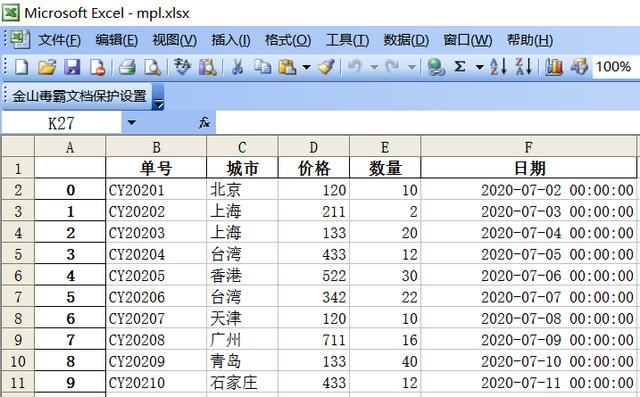折线图如下：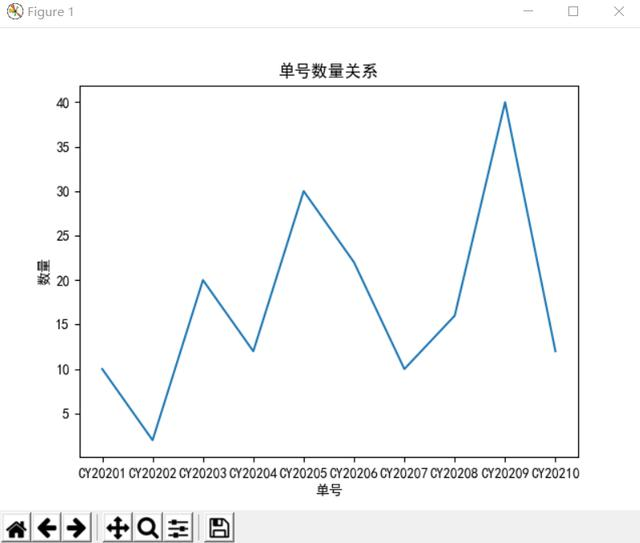条形图如下：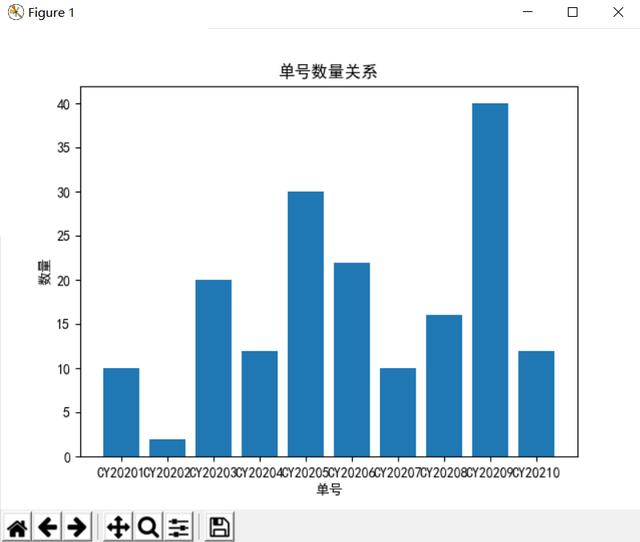饼状图如下：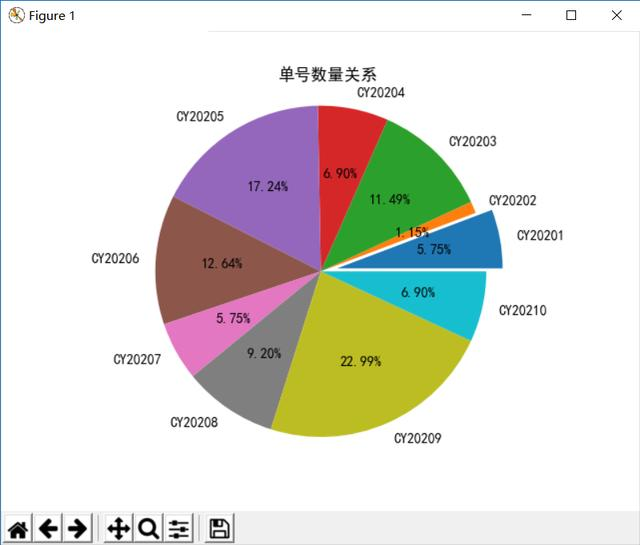展开全文可视化
• 安装数据可视化模块matplotlib:pip install ...1 折线图 from matplotlib import pyplot #横坐标 year=[2010,2012,2014,2016] #纵坐标 perple=[20,40,60,100] #生成折线图：函数polt pyplot.plot(year,perple)...matplotlib
• 这篇文章利用的是matplotlib.pyplot.plot的工具来绘制折线图，这里先给出一个段代码和结果图： # -*- coding: UTF-8 -*- import numpy as np import matplotlib as mpl import matplotlib.pyplot as plt #这里导入...
• Python-pyecharts折线图数据显示的问题 安装模块：xlrd (2.0.1)，pyecharts (1.9.0) 代码如下 import xlrd from pyecharts.charts import Line # 打开存储数据的excel data = xlrd.open_workbook('grzb.xls')...
• 主要为大家详细介绍了python使用matplotlib模块绘制多条折线图、散点图的方法，具有一定的参考价值，感兴趣的小伙伴们可以参考一下
• 使用Python绘制折线图的方法如下： import matplotlib.pyplot as plt import numpy as np #把三个折线放在同一张图内比较 acc1 = [[0.853,0.5865,0.6125,0.58,0.5675],[0.473333,0.33,0.23,0.25,0.26],[0.715556...errorbar
• 折线图： 也称为曲线图，它是用直线段将各数据点连接起来而组成的图形，以折线方式显示数据的变化趋势 1 折线图绘图函数： plot（x,y,style,color,linewidth） title('图的标题') 参数说明： style，划线的样式...
• 当我们获取完数据之后，一般来说数据可视化呈现的最基础图形就是：柱状图、水平条形图、折线图等等，在python的matplotlib库中分别可用bar、barh、plot函数来构建它们，再使用xticks与yticks（设置坐标轴刻度）、...
• 在谈及数据可视化的时候，我们通常都会使用到matplotlylib，pyecharts这些可视化的手段。但是，今天我主要来介绍Plotly这款可视化的库。大家参考开源项目地址：https://github.com/plotly/plotly.js这个库是使用js写...
• 以下文章来源于python数据分析之禅 ，作者鸟哥折线图是排列在工作表的列或行中的数据可以绘制到折线图中。折线图可以显示随时间（根据常用比例设置）而变化的连续数据，因此非常适用于显示在相等时间间隔下数据的...
• 1.Python通过曲线实时显示数据 代码： import matplotlib.pyplot as plt x = [] y = [] plt.ion() for i in range(100): x.append(i) y.append(i*i) plt.clf() plt.plot(x,y) plt.pause(0.01) plt.ioff()
• serif'] = ['SimHei'] # 用来正常显示中文标签 plt.ylabel(u'纵坐标') plt.xlabel(u'横坐标') A,=plt.plot(x4, y1, "bo-", linewidth=2, markersize=12, label=u"曲线一") B,=plt.plot(x4, y2, "gs-", linewidth=2, ...
• 接下来仅使用Python中的matplotlib模块和pandas模块实现折线图的绘制。尽管seaborn模块中的tsplot函数也可以绘制时间序列的折线图，但是该函数非常不合理，故不进行介绍。 1．matplotlib模块 折线图的绘制可以使用...
• 如果两个都安装成功了，还是显示matplotlib没有pyplot 这个类，那么就可以在pycharm里面尝试了，或者是安装2.2.1版本的matplotlib pip install matplotlib==2.2.1 下面就是代码了 import matplotlib.pyplot as plt x...
• practice.png双y轴折线图(plot both of those plots in one plot with 2 y-axislabels)一个y轴用来展示每年选秀总人数，另一个y轴用来展示赢球贡献值的...本文内容为重复 learning python:part 2 -...
• #功能: 导入4份文件(分别对应4个部位)，测算出其中2条生产线,4个部位的“平均库存时间”,以折线图方式显示 效果如下： 主程序为(Tl_B03.py)： import numpy as np import os import matplotlib.pyplot as plt ...
• #如果列表a便是10点到12点的每一分钟的气温，绘制折线图 # a=[random.randint(20,35)for i in range(120)] #解决中文不显示问题 #fc-list -->查看支持的字体 #fc-list :lang=zh -->查看支持的中文（冒号前有...
• 本人在学习使用Python和plotly处理数据时，经过两个小时艰难试错，终于完成了散点图和折线图的实例。在使用过程中遇到一个大坑，因为官方给出的案例是用在线存储的，所以需要安装jupyter（也就是ipython）才能使用...
• 数据可视化示例对数据可视化的浅认知数据可视化是任何数据科学或机器学习的重要组成部分。可视化能将数据以更加直观的...选择工具：matplotlibPython数据可视化最流行的工具之一是matplotlib，它是一个数学绘图库，...
• python matplotlib 画两条折线图 其中一组数据有空值 如何处理？ import csv from datetime import datetime from matplotlib import pyplot as plt filename = 'data.csv' with open(filename) as f: ...
• 说起折线图，很多人都觉得非常简单，不就是一些点连成的线吗？...如何对折线图进行数据分析？下面我们用一个具体的案例，演示折线图的分析过程和画图的方法。一个案例根据某公司 2019 年 9 月份每天的销量数据，...
• 1.画最简单的直线代码如下：import numpy as npimport matplotlib.pyplot as pltx=[0,1]y=[0,1]plt.figure()plt.plot(x,y)plt.savefig("easyplot.jpg")结果如下：代码解释：#x轴，y轴x=[0,1]y=[0,1]#...
• python折线图利用的是matplotlib.pyplot.plot的工具来绘制折线图，这里先给出一个段代码和结果图：# -*- coding: UTF-8 -*-import numpy as npimport matplotlib as mplimport matplotlib.pyplot as plt#这里导入...
• python折线图利用的是matplotlib.pyplot.plot的工具来绘制折线图，这里先给出一个段代码和结果图：# -*- coding: UTF-8 -*-import numpy as npimport matplotlib as mplimport matplotlib.pyplot as plt#这里导入...
• https://matplotlib.org/（查看使用matplotlib可制作的各种图表以及生成...
• 'w', newline='') as csvfile: writer = csv.writer(csvfile) for row in datas: writer.writerow(row) 生成折线图 import matplotlib.pyplot as plt import numpy as np import matplotlib.pyplot as plt plt....
• 主要用于开发2D图表，以渐进式、交互式的方式实现数据可视化，可以更直观的呈现数据，使数据更具说服力。 一、安装matplotlib pip install matplotlib -i https://pypi.tuna.tsinghua.edu.cn/simple 二、matplotlib......

# python折线图显示数据python 订阅